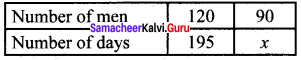# Samacheer Kalvi 8th Maths Solutions Term 3 Chapter 2 Life Mathematics Additional Questions

Students can Download Maths Chapter 2 Life Mathematics Additional Questions and Answers, Notes Pdf, Samacheer Kalvi 8th Maths Book Solutions Guide Pdf helps you to revise the complete Tamilnadu State Board New Syllabus and score more marks in your examinations.

## Tamilnadu Samacheer Kalvi 8th Maths Solutions Term 3 Chapter 2 Life Mathematics Additional Questions

Question 1.
120 men had food for 200 days. After 5 days 30 men left the camp. How long will the remaining food last.
Solution:
Since 30 men left after 5 days, the remaining food is sufficient for 120 men for 195 days. Suppose the remaining food lasts for x days for the remaining 90 men.
We haveMore men means less days the food lasts
∴ It is inverse proportion
120 : 90 = x : 195
Product of extremes = Product of means
120 × 195 = 90 × x
x = $$\frac{120×195}{90}$$
x = 90
x = 260.
∴ Remaining food last for 260 days.Question 2.
15 men earn Rs 900 in 5 days, how much will 20 men earn in 7 days?
Solution:
In one day 15 men earn Rs 900
In one day 15 men earn $$\frac{900}{5}$$ = Rs 180
In one day 1 men earn $$\frac{180}{5}$$ = Rs 12
∴ 1 men earn in 7 days = 12 × 7 = Rs 84
∴ 20 men earn in 7 days = 84 × 20 = 1680

Question 3.
A and B together can do a piece of work in 10 days, B and C can do the same work together in 12 days, A and C can do together in 15 days. How long will it take to complete the work working three of them altogether?
Solution:
(A + B)’s 1 day’s work = $$\frac{1}{10}$$……….(1)
(B + C)’s 1 day’s work = $$\frac{1}{12}$$……….(2)
(A + C)’s 1 day’s work = $$\frac{1}{15}$$……….(3)
(l) + (2) + (3) ⇒
[A + B + B + C + A + C]’s 1 day work = $$\frac{1}{10}$$ + $$\frac{1}{12}$$ + $$\frac{1}{15}$$
(2A + 2B + 2C)’s 1 day work = $$\frac{6 + 5 + 4}{60}$$
2(A + B + C)’s 1 day work = $$\frac{15}{60}$$
(A + B + C)’s 1 day’s work = $$\frac{1}{4 × 2}$$ = $$\frac{1}{8}$$
∴ A + B + C work together to finish the work in 8 days.